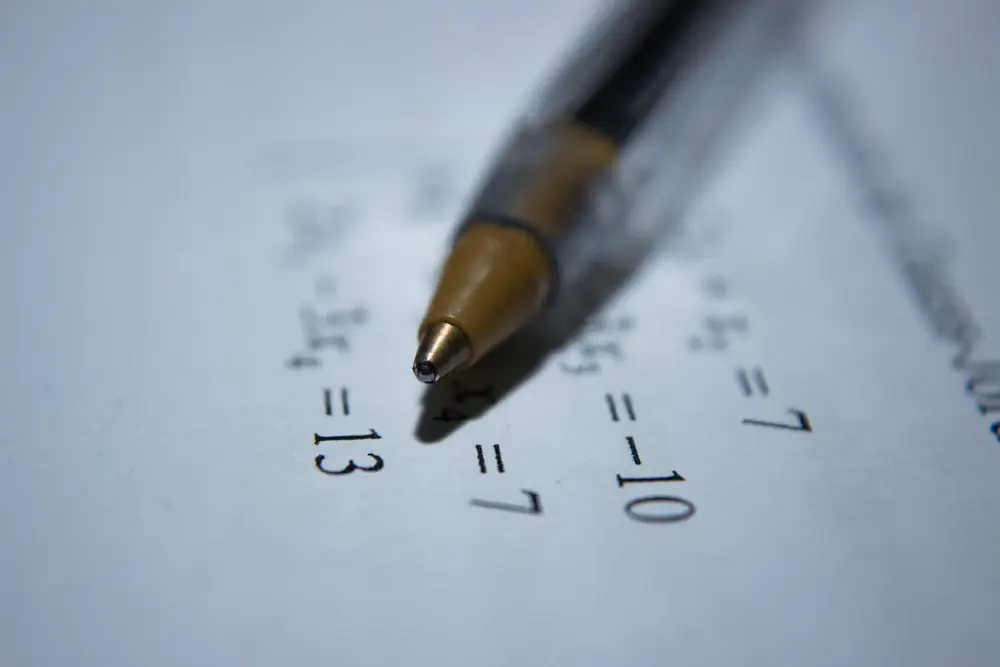# Set theory

Introduction
A set is a group of objects. Each object is known as as a member of the set. A set can be represented using curly brackets. So a set containing the numbers 2, 4, 6, 8, 10, ... is: {2, 4, 6, 8, 10, ... } . Sets are often also represented by letters, so this set might be E = {2, 4, 6, 8, 10, ...} . Alternatively, E = {even numbers} .

Common Sets
Some sets are commonly used and so have special notation:

Other Notation
If A = {1, 2, 4, 8} . n(A) = 4. This is because n(A) means the number of members in set A. The universal set is the set of all sets. All sets are therefore subsets of the universal set.

Venn Diagrams
Venn diagrams are used to represent sets. Here, the set A{1, 2, 4, 8} is shown using a circle. In Venn diagrams, sets are usually represented using circles. The universal set is the rectangle. The set A is therefore a subset of the universal set.

The complement of A, written A', contains all events in the sample space which are not members of A. A and A' together cover every possible eventuality.

AuB means the union of sets A and B and contains all of the elements of both A and B. This can be represented on a Venn Diagram as follows:

AnB means the intersection of sets A and B. This contains all of the elements which are in both A and B. AnB is shown on the Venn Diagram below:

Clearly n(A) + n(B) - n(AnB) = n(AuB)

Algebra

Algebra

Algebra

Algebra

Algebra

Algebra